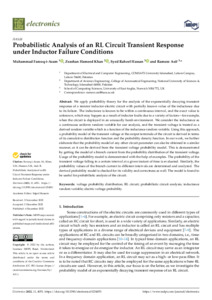# Probabilistic Analysis of an RL Circuit Transient Response under Inductor Failure Conditions

Farooq-i-Azam, Muhammad, Khan, Zeashan Hameed, Hassan, Syed Raheel and Asif, Rameez (2022) Probabilistic Analysis of an RL Circuit Transient Response under Inductor Failure Conditions. Electronics, 11 (23). ISSN 2079-9292Preview PDF (electronics-11-04051) - Published Version Available under License Creative Commons Attribution. Download (886kB) | Preview

## Abstract

We apply probability theory for the analysis of the exponentially decaying transient response of a resistor inductor electric circuit with partially known value of the inductance due to its failure. The inductance is known to be within a continuous interval, and the exact value is unknown, which may happen as a result of inductor faults due to a variety of factors—for example, when the circuit is deployed in an unusually harsh environment. We consider the inductance as a continuous uniform random variable for our analysis, and the transient voltage is treated as a derived random variable which is a function of the inductance random variable. Using this approach, a probability model of the transient voltage at the output terminals of the circuit is derived in terms of its cumulative distribution function and the probability density function. In our work, we further elaborate that the probability model of any other circuit parameter can also be obtained in a similar manner, or it can be derived from the transient voltage probability model. This is demonstrated by getting the model of a branch current from the probability distribution of the transient voltage. Usage of the probability model is demonstrated with the help of examples. The probability of the transient voltage falling in a certain interval at a given instant of time is evaluated. Similarly, the probability values of the branch current in different intervals are determined and analyzed. The derived probability model is checked for its validity and correctness as well. The model is found to be useful for probabilistic analysis of the circuit.

Item Type: Article 3* ,/dk/atira/pure/researchoutput/REFrank/3_ Faculty of Science > School of Computing Sciences Faculty of Science > Research Groups > Smart Emerging TechnologiesFaculty of Science > Research Groups > Cyber Security Privacy and Trust Laboratory LivePure Connector 07 Mar 2023 18:30 03 Sep 2023 01:18 https://ueaeprints.uea.ac.uk/id/eprint/91440 10.3390/electronics11234051View Item